# GUTS software ring test for the GUTS-package

## For performance reasons, this vignette builds from pre-calculated data.
##
##  To run all calculations, set 'do.calc <- TRUE' in the vignette's first code chunk.
##  Building the vignette will take a while.

# Summary

The GUTS package provides GUTS-RED variants GUTS-RED-IT, GUTS-RED-SD and GUTS-RED-Proper. For further information see Jager et al. (2011), Ashauer et al. (2016), Jager & Ashauer (2018) and EFSA PPR Panel (2018). The R-package implementation follows Albert et al. (2016), with minor enhancements.

This vignette demonstrates application of the individual tolerance model GUTS-RED-IT and the stochastic death model GUTS-RED-SD. The vignette is designed as verification test of model results. For this purpose, it runs one part of a recently conducted ring test on GUTS-software.

The ring test compared results from several software implementations of GUTS (Jager & Ashauer, 2018, Ch. 7). It focused on GUTS-RED-IT and GUTS-RED-SD models. Here, we follow the protocol of ring-test A, and perform the calibration as well as a forecast analysis.

# Prepare

library(GUTS)
packageVersion("GUTS")
#>  '1.2.3'

The ring test data was downloaded from http://www.debtox.info/book_guts.html and the data sheet with ring test A data is stored in file “Data_for_GUTS_software_ring_test_A_v05.xlsx” in the GUTS package. We recommend opening the file for data inspection.

# Data set A - synthetic data

This theoretical study assumed a chronic test with abundance measurements taken at exposure times. The synthetic data sets were generated with a GUTS-SD and a GUTS-IT model, using predefined parameters (Jager & Ashauer, 2018, Ch. 7.1.2, tab. 7.1).

Parameter values are:

par_A <- data.frame(symbols = c("hb", "ke", "kk", "mn", "beta"), JAsymbols = c("hb", "kd", "kk", "mw", "beta"), SD = c(0.01, 0.8, 0.6, 3, NA), IT = c(0.02, 0.8, NA, 5, 5.3))
par_A
#>   symbols JAsymbols   SD   IT
#> 1      hb        hb 0.01 0.02
#> 2      ke        kd 0.80 0.80
#> 3      kk        kk 0.60   NA
#> 4      mn        mw 3.00 5.00
#> 5    beta      beta   NA 5.30

Symbols refer to parameter symbols used in the GUTS package. JAsymbols denote the symbols used in Jager & Ashauer (2018).

## Synthetic data set from SD-model

The ring test data set A-SD is read from file “Data_for_GUTS_software_ring_test_A_v05.xlsx”.

The respective data table is in wide format. Here is the display as an R data.frame:

library(xlsx)
file = paste0(JA_data_file_name),
sheetName = "Data A",
rowIndex = c(1:11),
colIndex = seq(which(LETTERS == "A"), which(LETTERS == "G")),
)
#>    Table.1..Synthetic.survival.data.for.calibration.of.GUTS.SIC.SD.
#> 1                                                              <NA>
#> 2                                                              Time
#> 3                                                               Day
#> 4                                                                 0
#> 5                                                                 1
#> 6                                                                 2
#> 7                                                                 3
#> 8                                                                 4
#> 9                                                                 5
#> 10                                                                6
#>                              NA. NA..1 NA..2 NA..3 NA..4 NA..5
#> 1      Number of alive organisms    NA    NA    NA    NA    NA
#> 2  Concentration in micromol / L    NA    NA    NA    NA    NA
#> 3                              0     2     4     6     8    16
#> 4                             20    20    20    20    20    20
#> 5                             20    20    20    20    18     5
#> 6                             20    20    19    11     4     0
#> 7                             20    20    15     2     1     0
#> 8                             19    20    11     0     0     0
#> 9                             19    20     5     0     0     0
#> 10                            18    20     3     0     0     0

Extraction of the relevant data:

data_A_SD <- read.xlsx(
file = paste0(JA_data_file_name),
sheetName = "Data A",
rowIndex = c(4:11),
colIndex = seq(which(LETTERS == "B"), which(LETTERS == "G")),
)
con_A_SD <- as.numeric(data_A_SD[1,])
data_A_SD <- data_A_SD[-1,]
#the first row contains the concentrations
# all subsequent rows: number of alive organisms at specific day.

day_A_SD <-
as.numeric(
t(
file = paste0(JA_data_file_name),
sheetName = "Data A",
rowIndex = c(5:11),
colIndex = seq(which(LETTERS == "A")),
)
)
)

# name the data.frame
names(data_A_SD) <- paste0("c", con_A_SD)
rownames(data_A_SD) <- paste0("d", day_A_SD)

c: applied concentration; d: treatment day; each value indicates the number of alive organisms

## Set up the guts object

Setting up a GUTS-SD-object requires for each replicate of the treatment groups:

• the exposure profile containing the measured concentration C at each concentration measurement time Ct
• the survival data consisting of the number of survived organisms y at each abundance measurement time yt
• the GUTS-model type model = "SD"

For each replicate one GUTS object is created. All GUTS-objects are collected in a list. For the ring-test with one replicate for each of the 6 treatment groups, a list of 6 guts-objects is generated. We explicitly demonstrate how each GUTS-object in the list is constructed:

GUTS_A_SD <- list(
C0 = guts_setup(
C = rep_len(con_A_SD, length(day_A_SD)), Ct = day_A_SD,
y = data_A_SD$c0, yt = day_A_SD, model = "SD" ), C2 = guts_setup( C = rep_len(con_A_SD, length(day_A_SD)), Ct = day_A_SD, y = data_A_SD$c2, yt = day_A_SD,
model = "SD"
),
C4 = guts_setup(
C = rep_len(con_A_SD, length(day_A_SD)), Ct = day_A_SD,
y = data_A_SD$c4, yt = day_A_SD, model = "SD" ), C6 = guts_setup( C = rep_len(con_A_SD, length(day_A_SD)), Ct = day_A_SD, y = data_A_SD$c6, yt = day_A_SD,
model = "SD"
),
C8 = guts_setup(
C = rep_len(con_A_SD, length(day_A_SD)), Ct = day_A_SD,
y = data_A_SD$c8, yt = day_A_SD, model = "SD" ), C16 = guts_setup( C = rep_len(con_A_SD, length(day_A_SD)), Ct = day_A_SD, y = data_A_SD$c16, yt = day_A_SD,
model = "SD"
)
)

## Estimating parameters

Parameter estimation is conducted following suggestions Albert et al. (2016) and Supplementary material “S1: GUTS example R script”. For demonstration purposes in this vignette, the calibration procedure has been simplified and adjusted to the ring-test data set.

library('adaptMCMC') # Function MCMC(), Monte Carlo Markov Chain.

### Define joint log likelihood

The list of GUTS-objects is used to calculate the joint likelihood.

logposterior <- function( pars, guts_objects,
isOutOfBoundsFun = function(p) any( is.na(p), is.infinite(p) )  ) {
if ( isOutOfBoundsFun(pars) ) return(-Inf)
return(
sum(sapply( guts_objects, function(obj) guts_calc_loglikelihood(obj, pars) ))
)
}

### Defining constraints on parameter values

The logposterior function is formulated with a function that defines parameter bounds.

Constraints on parameters should consider

• hard boundaries:
• is.infinite(p) constrains parameters to finite values
• p<0 confines rates and thresholds to positive values
• knowledge on numerical limitations:
• p["kk"] > 30 confines the killing rate to avoid divergent Markov chains (see Albert et al., 2016).
• independent toxicological or ecological information: not used here
is_out_of_bounds_fun_SD <- function(p) any( is.na(p), is.infinite(p), p < 0, p["kk"] > 30 )

### Bayesian parameter estimation

Parameter values are estimated applying an adaptive Markov Chain Monte Carlo (MCMC) algorithm.

pars_start_SD <- rep_len (0.5, 4)
names(pars_start_SD) <- par_A$JAsymbols[-which(is.na(par_A$SD))]

mcmc_result_SD <- MCMC(p = logposterior,
init = pars_start_SD, adapt = 5000, acc.rate = 0.4, n = 150000,
guts_objects = GUTS_A_SD,
isOutOfBoundsFun = is_out_of_bounds_fun_SD
)

#exclude burnin and thin by 3
mcmc_result_SD$samples <- mcmc_result_SD$samples[seq(50001, 150000, by = 20),]
mcmc_result_SD$log.p <- mcmc_result_SD$log.p[seq(50001, 150000, by = 20)]

### Chain and distribution plots.

The top four graphs show mixing and distribution of the parameters. The last row “LL” shows mixing and distribution of the logposterior.


if (all(is.finite(mcmc_result_SD$log.p))) { par( mfrow = c(dim(mcmc_result_SD$samples) + 1, 2) , mar = c(5,4,1,0.5))
plot(as.mcmc(cbind(mcmc_result_SD$samples, LL = mcmc_result_SD$log.p)), auto.layout = FALSE)
par(op)
} else {
par( mfrow = c(dim(mcmc_result_SD$samples), 2) , mar = c(5,4,1,0.5)) plot(as.mcmc(mcmc_result_SD$samples), auto.layout = FALSE)
par(op)
}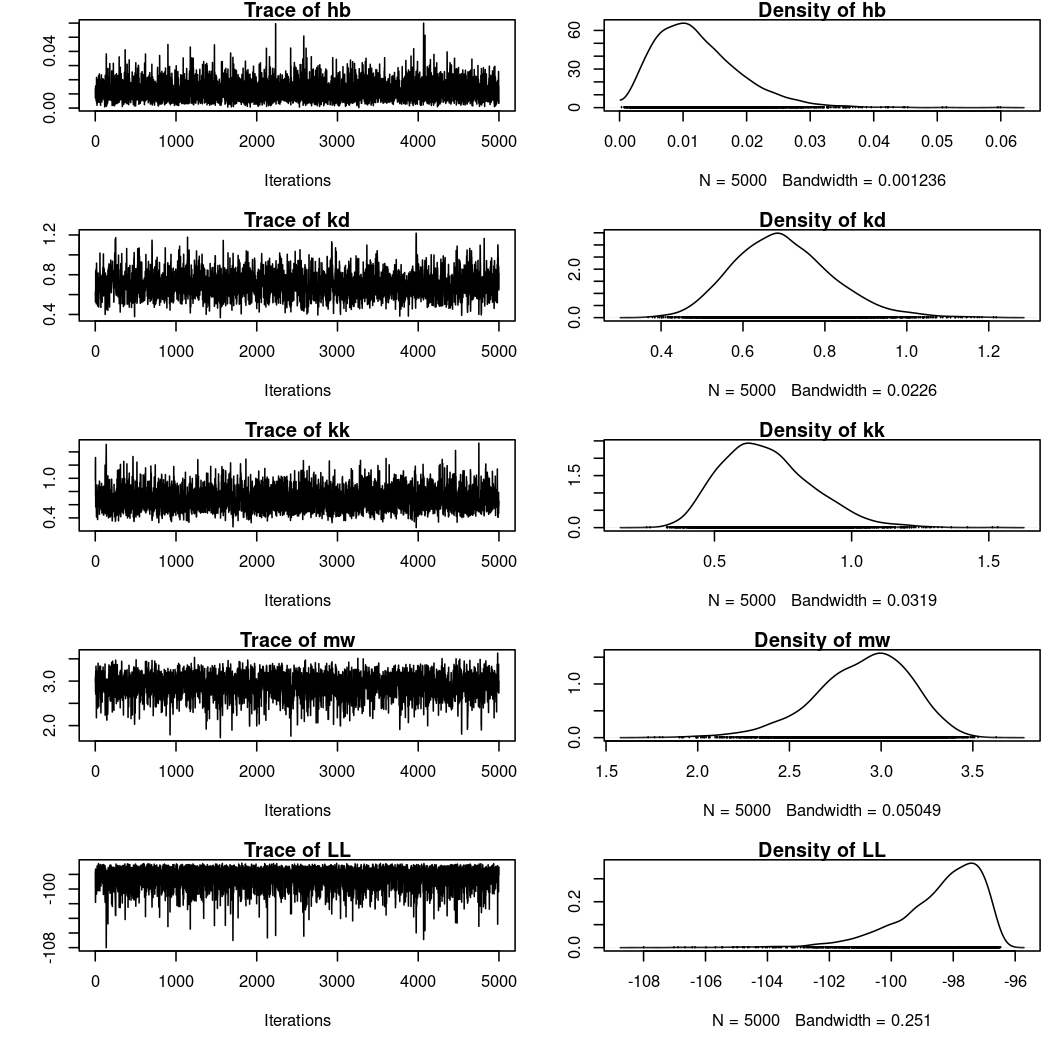### Estimated parameter values compared to the values that are used for data simulation in the ring test.

#### A plotting and evaluation function

This function takes a calibrated MCMC sample and summarizes the posterior distributions of the calibrated parameters. Calculated are for each calibrated parameter the value with highest posterior, as well as the median, the 2.5% and 97.5% quantiles of the posterior distribution. With the optional parameter expectedVal original values from the ring test can be given to compare them with the calibrated values. A graphical summary is plotted, if plot = TRUE.

eval_MCMC <- function(sampMCMC, expectedVal = NULL, plot = TRUE) {
bestFit <- sampMCMC$samples[which.max(sampMCMC$log.p),]
qu <- apply(sampMCMC$samples, 2, quantile, probs = c(0.025, 0.5, 0.975)) if (plot) { if(is.null(expectedVal)) expectedVal <- rep(NA, dim(sampMCMC$samples))
plot(seq(dim(sampMCMC$samples)), expectedVal, pch = 20, col = "darkgrey", cex = 2, ylim = range(qu), xaxt = "n", xlab = "Model parameter", ylab = "Parameter value") arrows(x0 = seq(dim(sampMCMC$samples)), y0 = qu[1,], y1 = qu[3,], angle = 90, length = 0.1, code = 3)
points(x = seq(dim(sampMCMC$samples)), y = bestFit, pch = "-", cex = 4) axis(side = 1, at = seq(dim(sampMCMC$samples)), dimnames(sampMCMC$samples)[]) } res <- rbind(bestFit, qu) rownames(res) <- "best" if (!all(is.na(expectedVal))) { res <- rbind(res, expectedVal) rownames(res)[dim(res)] <- "expect" } return(res) } #### Estimated parameters eval_MCMC(mcmc_result_SD, expectedVal = par_A$SD[-which(is.na(par_A$SD))])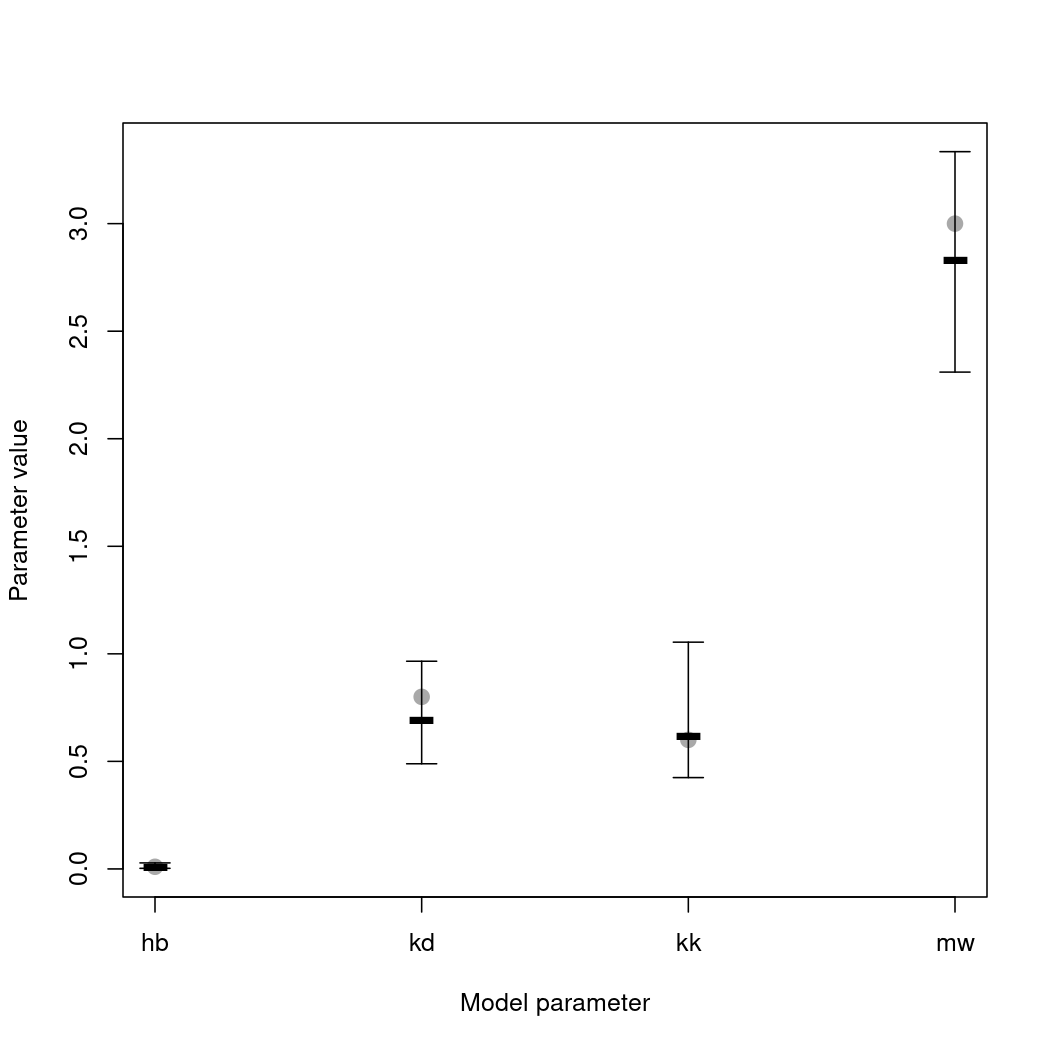#> hb kd kk mw #> best 0.007747013 0.6914421 0.6172954 2.831710 #> 2.5% 0.002763125 0.4888775 0.4246350 2.309847 #> 50% 0.011203457 0.6894394 0.6710864 2.927733 #> 97.5% 0.028080870 0.9656203 1.0542871 3.334637 #> expect 0.010000000 0.8000000 0.6000000 3.000000 Black lines indicate best estimates and the error bars the 95% credible interval; grey dots are the model parameter values used to generate the data for the ring test. ## Synthetic data set from IT-model The ring test data set A-IT is read from file “Data_for_GUTS_software_ring_test_A_v05.xlsx”. Extraction of the relevant data: data_A_IT <- read.xlsx( file = paste0(JA_data_file_name), sheetName = "Data A", rowIndex = c(17:24), colIndex = seq(which(LETTERS == "B"), which(LETTERS == "G")), header = FALSE ) con_A_IT <- as.numeric(data_A_IT[1,]) data_A_IT <- data_A_IT[-1,] #the first row contains the concentrations # all subsequent rows: number of alive organisms at specific day. day_A_IT <- as.numeric( t( read.xlsx( file = paste0(JA_data_file_name), sheetName = "Data A", rowIndex = c(18:24), colIndex = seq(which(LETTERS == "A")), header = FALSE ) ) ) # name the data.frame names(data_A_IT) <- paste0("c", con_A_IT) rownames(data_A_IT) <- paste0("d", day_A_IT) c: applied concentration; d: treatment day; each value indicates the number of alive organisms ## Set up the guts object Setting up a GUTS-IT-object requires for each replicate of the treatment groups: • the exposure profile containing the measured concentration C at each concentration measurement time Ct • the survival data consisting of the number of survived organisms y at each abundance measurement time yt • the GUTS-model type model = "IT" • the tolerance threshold distribution dist = "loglogistic", as log-logistic was used in the ring test. For each replicate one GUTS object is created. All GUTS-objects are collected in a list. For the ring-test with one replicate for each of the 6 treatment groups, a list of 6 guts-objects is generated. Here, we exemplarily show an automatized procedure to create the list of GUTS-objects from the data. GUTS_A_IT <- lapply(seq(length(con_A_IT)), function(i, dat, days, con) guts_setup( C = rep_len(con[i], length(days)), Ct = days, y = dat[,i], yt = days, model = "IT", dist = "loglogistic" ), dat = data_A_IT, days = day_A_IT, con = con_A_IT ) names(GUTS_A_IT) <- paste0("c", con_A_IT) ## Estimating parameters ### Defining constraints on parameter values The logposterior function is formulated with a function that defines parameter bounds. Constraints on parameters should consider • hard boundaries: • is.infinite(p) constrains parameters to finite values • p<0 confines rates and thresholds to positive values • knowledge on numerical limitations: • exp(8/p) * p > 1e200 avoids numerical problems when approximating the log-logistic function for unrealistically high median values p and extremely low shape values p that result in a threshold distribution confined at a zero threshold. • p <= 1 is assumed, to exclude that the mode of the log-logistic threshold distribution is 0, due to the shape parameter. Note that the mode can approach 0 if the estimated median p approaches 0. Therefore, this constraint stabilises estimation of low threshold values. • toxicological or ecological information: not used here is_out_of_bounds_fun_IT <- function(p) any( is.na(p), is.infinite(p), p < 0, p <= 1, exp(8/p) * p > 1e200) ### Bayesian parameter estimation Parameter values are estimated applying an adaptive Markov Chain Monte Carlo (MCMC) algorithm. pars_start_IT <- rep_len(0.5, 4) names(pars_start_IT) <- par_A$JAsymbols[-which(is.na(par_A$IT))] mcmc_result_IT <- MCMC(p = logposterior, init = pars_start_IT, adapt = 5000, acc.rate = 0.4, n = 150000, guts_objects = GUTS_A_IT, isOutOfBoundsFun = is_out_of_bounds_fun_IT ) #exclude burnin and thin by 3 mcmc_result_IT$samples <- mcmc_result_IT$samples[seq(50001, 150000, by = 20), ] mcmc_result_IT$log.p <- mcmc_result_IT$log.p[seq(50001, 150000, by = 20)] #### Chain and distribution plots The top four graphs show mixing and distribution of the parameters. The last row “LL” shows mixing and distribution of the logposterior. if (all(is.finite(mcmc_result_IT$log.p))) {
par( mfrow = c(dim(mcmc_result_IT$samples) + 1, 2) , mar = c(5,4,1,0.5)) plot(as.mcmc(cbind(mcmc_result_IT$samples, LL = mcmc_result_IT$log.p)), auto.layout = FALSE) par(op) } else { par( mfrow = c(dim(mcmc_result_IT$samples), 2) , mar = c(5,4,1,0.5))
plot(as.mcmc(mcmc_result_IT$samples), auto.layout = FALSE) par(op) }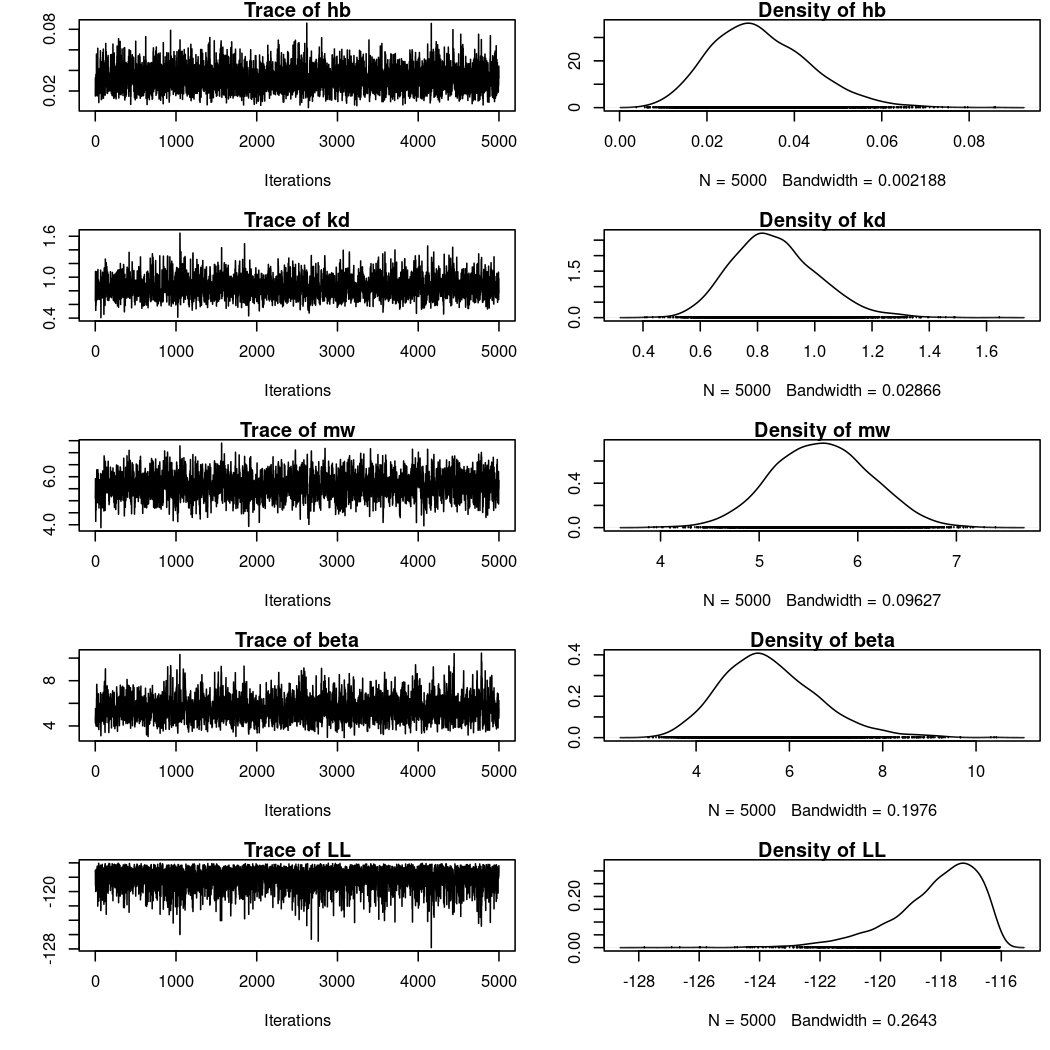### Estimated parameter values compared to the values that were used for data simulation. eval_MCMC(mcmc_result_IT, expectedVal = par_A$IT[-which(is.na(par_A$IT))])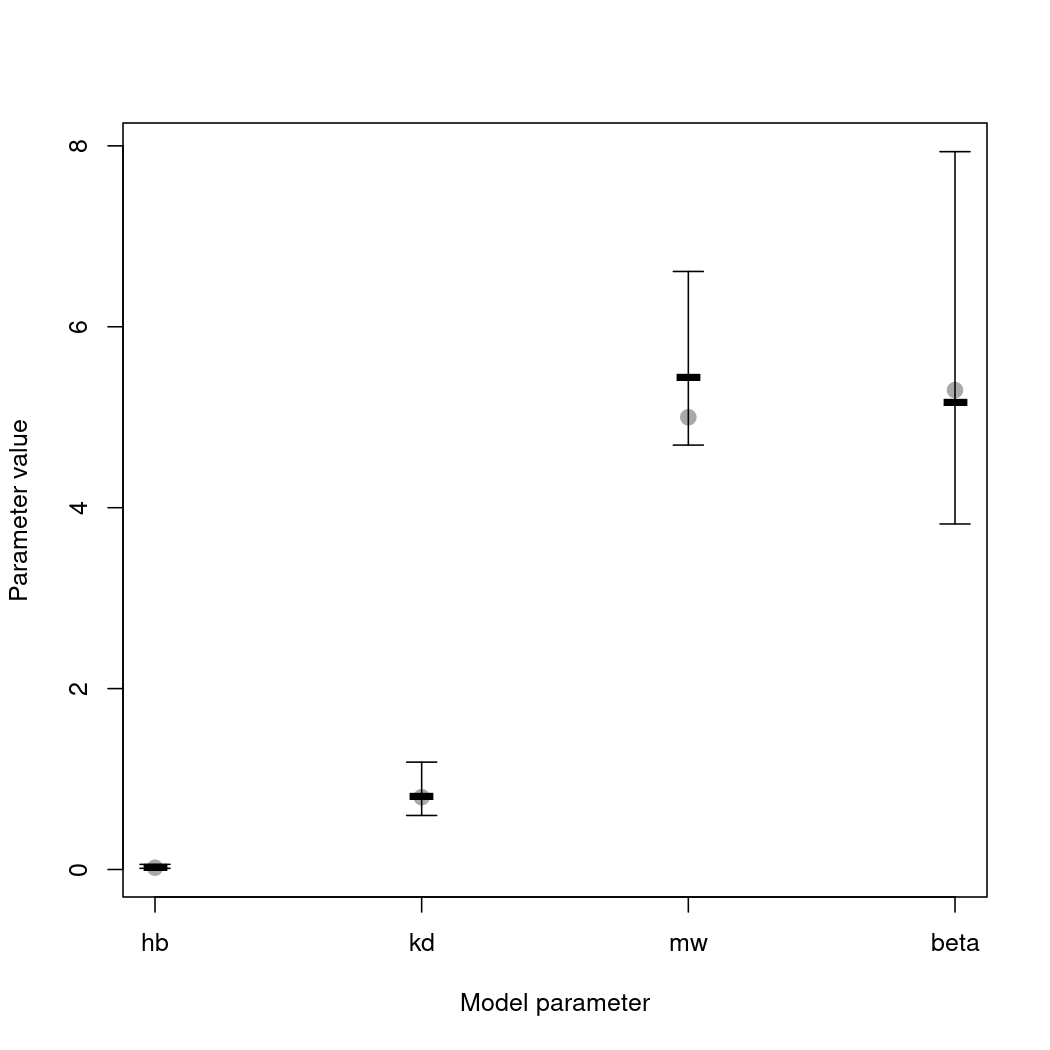#> hb kd mw beta #> best 0.02707007 0.8127029 5.448312 5.168084 #> 2.5% 0.01308660 0.5975469 4.691963 3.819837 #> 50% 0.03115691 0.8481939 5.631914 5.470063 #> 97.5% 0.05698634 1.1869923 6.611356 7.935804 #> expect 0.02000000 0.8000000 5.000000 5.300000 Black lines indicate best estimates and the error bars the 95% credible interval; grey dots are the model parameter values used to generate the data for the ring test. # Forecast The GUTS-package can be applied to forecast survival, too. We demonstrate this feature for the calculation of a 4d-LC50 value assuming constant exposure. ## Setup guts object and forecast Values to be specified are • concentrations C • concentration time points Ct • number of individuals y • length(y) corresponds to the number of time steps for which predictions are required. • y specifies the initial number of individuals. • other entries of y can be chosen arbitrarily and will not be used. • forecast time points yt: a vector starting with 0 To calculate the LC50, we use the GUTS object to simulate the survival during a 4 day period for several constant dose levels. Here, we uses doses 0 to 16 in steps of 2. We choose 6 observation and application time steps for illustration purposes. In fact, to calculate 4d-LC50, it would be sufficient to specify the initial number of individuals at time step 0, and specify time step 4 days as the observation time. We assume an initial population size of 100 individuals. conc <- seq(0, 16, by = 2) guts_obj_forecast <- lapply(conc, function(concentration) guts_setup( C = rep(concentration, 7), Ct = seq(0,12, by = 2), y = c(100, rep(0,6)), yt = seq(0,12, by = 2), model = "IT", dist = "loglogistic", N = 1000 ) ) We forecast survival probabilities for each dose concentration and the parameter sets in the posterior distribution. This procedure takes into account uncertainties in parameter estimates. According to the protocol for the ring test, for prediction the background mortality “hb” was fixed at 0, in order to isolate the treatment effects in model forecasts. mcmc_forecasts_paras <- mcmc_result_IT$samples
mcmc_forecasts_paras[,1] <- 0
forec <- lapply(guts_obj_forecast,
function(gobj, mcmc_res)
rbind(
rep(gobj$C, dim(mcmc_forecasts_paras)), apply(mcmc_res, 1, function(pars) guts_calc_survivalprobs(gobj = gobj, pars, external_dist = NULL) ) ), mcmc_res = mcmc_forecasts_paras ) forec <- do.call("cbind", forec) forec <- as.data.frame(t(forec)) names(forec) <- c("conc", paste0("day", guts_obj_forecast[]$yt))

## Analyse projections

The box-whisker-plots show dose-response relationships as forecasted for the specific days.

par(mfrow = c(2,3), mar = c(5,4,3, 0.5))
sapply(tail(names(forec), -2),
function(day)
plot(as.factor(forec\$conc), forec[, day],
ylim = c(0,1),
xlab = "concentration (micromol/l)", ylab = "probability of survival",
main = day)
)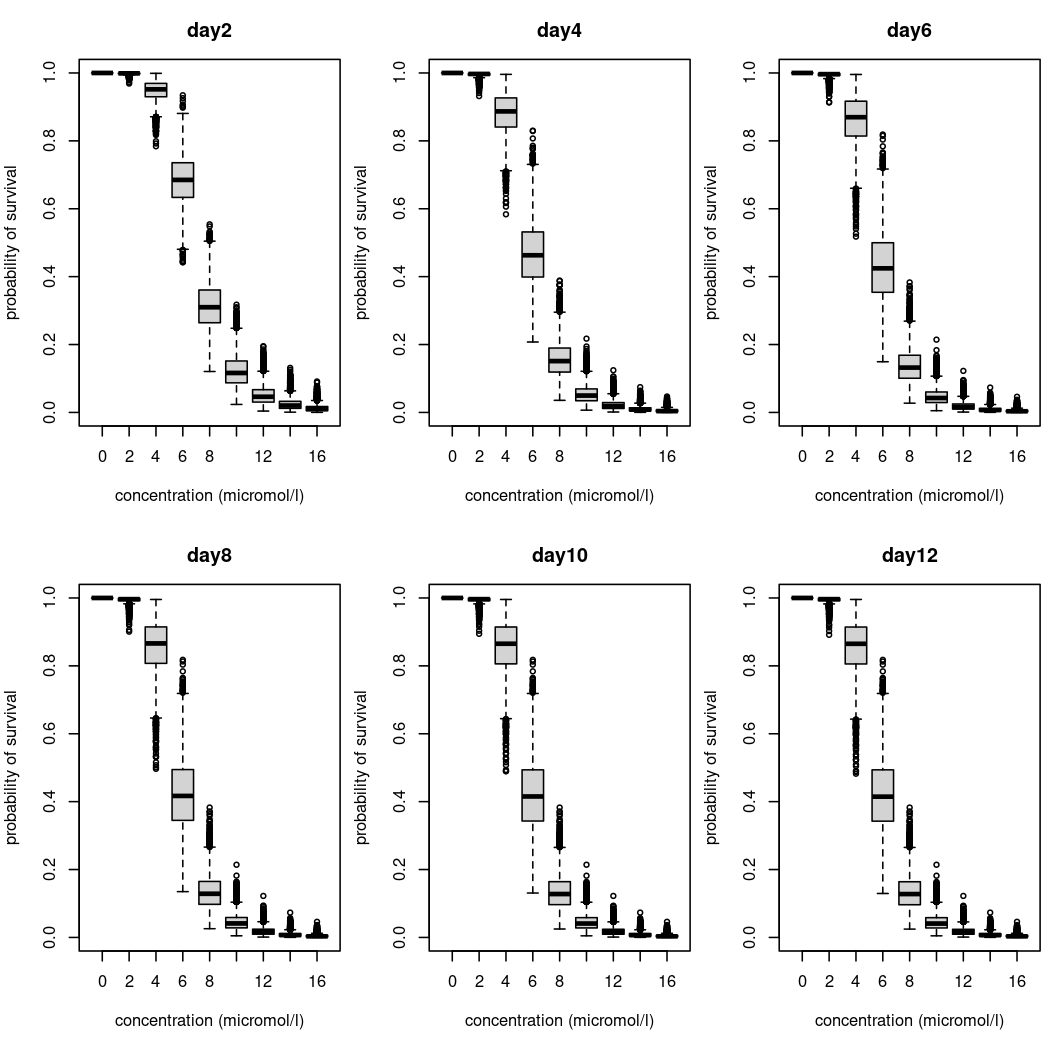par(op)

For example, the drc-package (Ritz et al. 2015) can be applied to estimate the d4-LD50. A log-logistic dose-response interpolation is assumed. By estimating the LD50 for each sampled parameter set separately, the uncertainty in LC50 estimates can be derived from the parameter uncertainty revealed by the Bayesian analysis.

library("drc")
logLC50s <- sapply(seq_len(dim(mcmc_forecasts_paras)),
function(indParaset, forDat, concentrations) {
dat <- forDat[dim(mcmc_forecasts_paras) * (seq_along(concentrations) - 1) + indParaset,"day4"]
return(
coefficients(
drm(data.frame(dat, concentrations), fct = LL2.3(names = c("Slope", "upper", "logLC50")))
)
)
},
forDat <- forec,
concentrations = conc
)
LC50 <- quantile(exp(logLC50s), c(0.025, 0.5, 0.975))

The estimated LC50 median of 5.8 (CI = [5; 6.7]) is in accordance with the ring test forecast (Fig. 7.5, Data-A forecast 4d-LC50-IT in Jager & Ashauer, 2018).

# Literature

Albert, C., Vogel, S., and Ashauer, R. (2016). Computationally efficient implementation of a novel algorithm for the General Unified Threshold Model of Survival (GUTS). PLOS Computational Biology, 12(6), e1004978. doi: 10.1371/journal.pcbi.1004978.

Ashauer, R., Albert, C., Augustine, S., Cedergreen, N., Charles, S., Ducrot, V., Focks, A., Gabsi, F., Gergs, A., Goussen, B., Jager, T., Kramer, N.I., Nyman, A.-M., Poulsen, V., Reichenberger, S., Schäfer, R.B., Van den Brink, P.J., Veltman, K., Vogel, S., Zimmer, E.I., Preuss, T.G. (2016). Modelling survival: exposure pattern, species sensitivity and uncertainty. Scientific Reports, 6, 1, doi: 10.1038/srep29178

Jager, T., Albert, C., Preuss, T., and Ashauer, R. (2011). General Unified Threshold Model of Survival - a toxicokinetic toxicodynamic framework for ecotoxicology. Environmental Science & Technology, 45(7), 2529-2540, doi: 10.1021/es103092a.

Jager, T., Ashauer, R. (2018). Modelling survival under chemical stress. A comprehensive guide to the GUTS framework. Leanpub: https://leanpub.com/guts_book, http://www.debtox.info/book_guts.html.

EFSA PPR Panel (EFSA Panel on Plant Protection Products and their Residues), Ockleford, C., Adriaanse, P., Berny, P., Brock, T., Duquesne, S., Grilli, S., Hernandez-Jerez, A.F., Bennekou, S.H., Klein, M., Kuhl, T., Laskowski, R., Machera, K., Pelkonen, O., Pieper, S., Smith, R.H., Stemmer, M., Sundh, I., Tiktak, A., Topping, C.J., Wolterink, G., Cedergreen, N., Charles, S., Focks, A., Reed, M., Arena, M., Ippolito, A., Byers, H. and Teodorovic, I. (2018). Scientific Opinion on the state of the art of Toxicokinetic/Toxicodynamic (TKTD) effect models for regulatory risk assessment of pesticides for aquatic organisms. EFSA Journal, 16(8):5377, 188 pp., doi: 10.2903/j.efsa.2018.5377.

Ritz, C., Baty, F., Streibig, J. C., Gerhard, D. (2015). Dose-Response Analysis Using R. PLOS ONE, 10(12), e0146021, doi: 10.1371/journal.pone.0146021